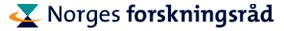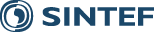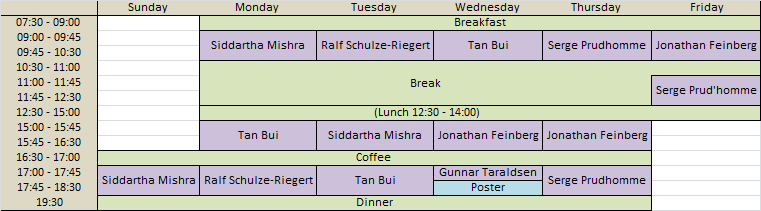Schedule and program

## At a glancePrinted program

## Lecture abstracts

### Tan Bui-Thanh (University of Texas)

#### Tutorial on Statistical Inversion and UQ using the Bayesian Paradigm

Inverse problems and uncertainty quantification (UQ) are ubiquitous in engineering and science, especially in scientific discovery and decision-making for complex scientific and societal problems. Though the past decades have seen tremendous advances in both theories and computational algorithms for inverse problems, quantifying the uncertainty in their solution is still enormously challenging, and hence a very active research. This lecture will cover various aspects of Bayesian inverse problems and UQ in a constructive and systematic manner: from probability theory, construction of priors, and construction of likelihoods to Markov chain Monte Carlo theory. If time permits, we will cover some contemporary topics such as scalable methods for large-scale Bayesian inverse problems with high dimensional parameter spaces and with big data.

The lecture is accompanied by matlab codes that can be used to reproduce most of results, demonstrations, and beyond.

### Jonathan Feinberg (Simula Research Laboratory)

#### Polynomial chaos expansions part I: Method Introduction

Polynomial chaos expansion is a class of methods for determining the uncertainties in forward models given uncertainty in the input parameters. It is a new method made popular in recent years because of its very fast convergence. In this first lecture we will introduce the general concepts necessary to understand the foundation of the theory. We will in parallel introduce Chaospy: a Python toolbox specifically developed to implement polynomial chaos expansions.

#### Polynomial chaos expansions part II: Practical Implementation

Implementing polynomial chaos expansions in practice comes with a few challenges. This lecture will teach how to address these. In particular we will be looking at non-intrusive methods where we can assume that the underlying model solver is a "black box". We will show how to use Chaospy on a large collection of problems, with a walk-through of the initial setup, implementation and analysis.

#### Polynomial chaos expansions part III: Some advanced topics

In some cases the basic theory of polynomial chaos expansion is not enough. For example, one fundamental assumption is that the input parameters are stochastically independent. In this lecture we will take a look at what can be done when this assumption no longer holds. Additionally we will look at intrusive polynomial chaos methods. Here we couple the polynomial chaos expansion directly to the set of governing equations, which results in a higher accuracy in the estimations.

### Siddartha Mishra (ETH Zurich)

#### UQ for nonlinear hyperbolic PDEs I.

I will introduce nonlinear hyperbolic systems of conservation laws and mention examples of models described by them. Essential concepts such as shocks, weak solutions and entropy conditions will be briefly reviewed. A short course on state of art numerical methods of the high-resolution finite volume type will be given. The challenges of modeling uncertainty in inputs and in the resulting
solutions will be motivated. The last part will consist of demonstration of some numerical experiments with ALSVID -- a C++/python based conservation laws solver.

#### UQ for nonlinear hyperbolic PDEs II

We describe statistical sampling methods for UQ for hyperbolic conservation laws. The Monte Carlo (MC) method is explained, corresponding error estimates derived and difficulties with this method illustrated. The Multi-level Monte Carlo (MLMC) method is introduced to make the computation much more efficient. This method is also described in terms of a user guide to implement it and many numerical experiments (using ALSVID-UQ) are shown to illustrate the method.

#### UQ for nonlinear hyperbolic PDEs III

We discuss some limitations of the MLMC method, particularly for systems of conservation laws. An alternative UQ framework, the so-called Measure valued and statistical solutions is introduced and discussed in some detail. Both MC and MLMC versions of efficient algorithms to compute Measure valued solutions are described. If time permits, we discuss some aspects of implementing MC and MLMC on massively parallel and hybrid architectures.

### Serge Prudhomme (Polytechnique Montréal)

#### A Review of Goal-oriented Error Estimation and Adaptive Methods

The topic of this lecture deals with the derivation of a posteriori error estimators to estimate and control discretization errors with respect to quantities of interest. The methodology is based on the notion of the adjoint problem and can be viewed as a generalization of the concept of Green's function used for the calculation of point-wise values of the solution of linear boundary-value problems. Moreover, we will show how to exploit the computable error estimators to derive refinement indicators for mesh adaptation. We will also generalize the error estimators in quantities of interest to the case of nonlinear boundary-value problems and to nonlinear quantities of interest. It will be shown that the methodology provides similar error estimators as those obtained in the linear case, except for linearization errors due to the linearization of the quantities of interest and of the adjoint problems.

#### Error Estimation and Control for Problems with Uncertain Coefficients

The objective of this lecture will be to present an approach for adaptive refinement with respect to both the physical and parameter discretizations in the case of boundary-value problems with uncertain coefficients. The approach relies on the ability to decompose the error into contributions from the physical discretization error and from the response surface approximation error in parameter space. The decomposition of the errors and adaptive technique will allows one to optimally use available resources to accurately estimate and quantify the uncertainty in a specific quantity of interest.

#### Adaptive Construction of Response Surface Approximations for Bayesian Inference

In this lecture, we extend our work on error decomposition and adaptive methods with respect to response surfaces to the construction of a surrogate model that can be utilized for the identification of parameters in Reynolds-averaged Navier-Stokes models. The error estimates and adaptive schemes are driven here by a quantity of interest and are thus based on the approximation of an adjoint problem. The desired tolerance in the error of the posterior distribution allows one to establish a threshold for the accuracy of the surrogate model.

### Ralf Schulze-Riegert (Schlumberger)

Optimization under uncertainty remains a challenging exercise in reservoir modelling across the oil and gas industry. "A strong positive correlation between the complexity and sophistication of an oil company's decision analysis and its financial performance exists. Underestimation of magnitude of reservoir uncertainties and their interdependencies can lead to sub optimal decision making and financial underperformance of any project." (SPE93280).
This session presents practical implementations of uncertainty quantification workflows using examples in reservoir management. Existing methods as well as emerging trends for new technologies used for estimating prediction uncertainties in reservoir simulation will be discussed.

#### Estimation of prediction uncertainties in reservoir simulation using Bayesian and proxy modelling techniques

Subsurface uncertainties have a large impact on oil & gas production forecasts. Underestimation of prediction uncertainties therefore presents a high risk to investment decisions for facility designs and exploration targets. The complexity and computational cost of reservoir simulation models often defines narrow limits for the number of simulation runs used in related uncertainty quantification studies.
In this session we will look into workflow designs and methods that have proven to deliver results in industrial reservoir simulation workflows. Combinations of automatic proxy modelling, Markov Chain Monte Carlo and Bayesian approaches for estimating prediction uncertainties are presented.

#### Integration requirements for cross-disciplinary uncertainty quantification turn workflows into a big-loop and big-data exercise?

Reservoir model validation and uncertainty quantification workflows have significantly developed over recent years. Different optimization approaches were introduced and requirements for consistent uncertainty quantification workflows changed. Most of all integration requirements across multiple domains (big-loop) increase the complexity of workflow designs and amount of data (big-data) processed in the course of workflow execution. This triggers new requirements for the choice of optimization and uncertainty quantification methods in order to add value to decision processes in reservoir management. In this session we will discuss an overview on existing methods and perspectives for new methodologies based on parameter screening, proxy-based as well as analytical sensitivity approaches.

### Gunnar Taraldsen

#### ISO GUM, Uncertainty Quantification, and Philosophy of Statistics

In 1978, recognizing the lack of international consensus on the expression of uncertainty in measurement, the world's highest authority in metrology, the Comité International des Poids et Mesures (CIPM), requested the Bureau International des Poids et Mesures (BIPM) to address the problem in conjunction with the national standards laboratories and to make a recommendation. As a result the International Organization for Standardization (ISO) published the first version of the Guide to the expression of Uncertainty of Measurements (GUM) in 1993. This session will discuss uncertainty quantification in relation to the ISO GUM, which states:
"Just as the nearly universal use of the International System of Units (SI) has brought coherence to all scientific and technological measurements, a worldwide consensus on the evaluation and expression of uncertainty in measurement would permit the significance of a vast spectrum of measurement results in science, engineering, commerce, industry, and regulation to be readily understood and properly interpreted. In this era of the global marketplace, it is imperative that the method for evaluating and expressing uncertainty be uniform throughout the world so that measurements performed in different countries can be easily compared."
Additionally, we will discuss the relation between uncertainty quantification and the philosophical foundations of statistics.

Published December 1, 2014